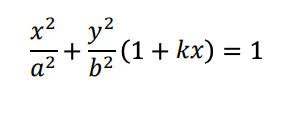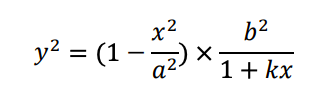Tricky Integration Question

topsquark

Senior Member
That's an integral over x, I presume?

-Dan

BigBeachBananas

Full Member
Why is there a 2y in the denominator? You have 3 variables k,x,y and 1 equation. Please post the full original question.

swal

New member
Why is there a 2y in the denominator? You have 3 variables k,x,y and 1 equation. Please post the full original question.
The function is a relation - I'm trying to find the length of the curve of this function:Where a is 2.95 and b is 2. I need the length of the curve between -2.95 and 2.95, given y is greater than or equal to zero.This can be rearranged as:This can be implicitly differentiated in order to substitute into the formula for the length of an arc, but I can't do the integration.

BigBeachBananas

Full Member
This can be implicitly differentiated in order to substitute into the formula for the length of an arc, but I can't do the integration.
Take the square root?
$y=\pm \sqrt{\left(1-\frac{x^2}{a^2}\right)\frac{b^2}{1+kx}}$
y is greater than or equal to zero
Take the positive only.
$y=\sqrt{\left(1-\frac{x^2}{a^2}\right)\frac{b^2}{1+kx}}$First, I would simplify then find the derivative. But it does not look pretty...

Last edited:

Dr.Peterson

Elite Member
The function is a relation - I'm trying to find the length of the curve of this function:
View attachment 30672
Where a is 2.95 and b is 2. I need the length of the curve between -2.95 and 2.95, given y is greater than or equal to zero.This can be rearranged as: View attachment 30673
This can be implicitly differentiated in order to substitute into the formula for the length of an arc, but I can't do the integration.
The integrand must be a function of x; implicit differentiation doesn't initially produce that, because you still have y in the derivative. You need to replace y with its expression in terms of x (BBB's radical) before integrating. (Assuming the rest of your work is correct, which I haven't looked at.)

Not all functions can be integrated exactly, or with a reasonable amount of work! This may well be one of the many that would need numerical integration.

BigBeachBananas

Full Member
I feel like you haven't posted the full question and its instruction. For the integral to converge, [imath]-0.3 \le k \le 0.3[/imath]. Notice for [imath]k=0[/imath], it's an ordinary ellipse. Was this info given? Are you expect to integrate by hand? You haven't given us the full context of the question.

BigBeachBananas

Full Member
ok, so I've done that. Can anyone integrate this with respect to x in order to find k?
View attachment 30688
I doubt even WolframAlpha can. You won't get any further until you show us the exact original question.

Jomo

Elite Member
I've always wondered why some posters insist on given the entire problem over a few posts.